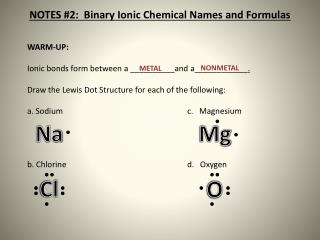DownloadDownload PresentationNOTES #2: Binary Ionic Chemical Names and Formulas

# NOTES #2: Binary Ionic Chemical Names and Formulas

Télécharger la présentation## NOTES #2: Binary Ionic Chemical Names and Formulas

- - - - - - - - - - - - - - - - - - - - - - - - - - - E N D - - - - - - - - - - - - - - - - - - - - - - - - - - -
##### Presentation Transcript

1. WARM-UP: • Ionic bonds form between a __________and a____________. • Draw the Lewis Dot Structure for each of the following: • a. Sodiumc. Magnesium • b. Chlorine d. Oxygen NONMETAL METAL NOTES #2: Binary Ionic Chemical Names and Formulas Na Mg Cl O

2. NOTES #2: Binary Ionic Chemical Names and Formulas

3. NOTES #2: Forming Ionic Compounds using the Crisscross Rule and Nonmetals! Label Metals Metals http://go.hrw.com/resources/go_sc/periodic/Po_At_Metalloids.pdf

4. 4- 3- 2- 1- NOTES #2 – Reviewing the Charges on Cations and Anions Transition Metals Vary – Will discuss later 3+ 4+ 1+ 2+

5. Important Facts about Ionic Compounds • The sum of the charges must add up to zero, so nocharges are written in the final formula of a compound. • MgF2 • We use subscripts to represent the number of each ion. • Al2O3 • 2 Aluminum Ions and 3 Oxide Ions • They are written as the lowest possible ratio. • Ca4F8 CaF2

6. Important Facts about Ionic Compounds • The overall charge on the compound must equal zero, that is, the number of electrons lost by one atom must equal the number of electrons gained by the other atom. • The Lewis Structure (electron dot diagram) of each ion is used to construct the Lewis Structure (electron dot diagram) for the ionic compound.

7. Important Facts about Ionic Compounds • Examples: Lithium fluoride, LiF • Lithium atom loses one electron to form the cation Li+ • Fluorine atom gains one electron to form the anion F- • Lithium fluoride compound can be represented as

8. Important Facts about Ionic Compounds • Examples: Lithium oxide • Lithium atom loses one electron to form the cation Li+ • Oxygen atom gains two electrons to form the anion O2-

9. Magnesium chloride

10. Remember the charge of an ion is determined by its valence electrons and how it obeys the octet rule! • Sum of charges must equal zero. Ex: • Na+1+ Cl-1 NaCl (+1 + -1 = 0) • Mg+2+ O-2 MgO * (+2 + -2 = 0) • *Don’t write this Mg2O2 because it must be reduced (THE TWOS CANCEL OUT). • The charges for each of these two sets of ions equals zero, so they are combined in a 1:1 ratio. NOTES #2: Forming Ionic Compounds using the Crisscross Rule

11. Independent Practice NOTES #2: Binary Ionic Chemical Names and Formulas

12. The Assessment on page 9 #1-3, Lewis Structure only on #3-11. • Study Polyatomic ions Pg. 12 and prefixes Pg. 17 for quiz Friday. NOTES #2: Assessment

13. Criss-Cross Rule! • If charges do not equal zero, use the crisscross rule (shown on the next slide). • The charge number (absolute value) becomes subscript of the other element. This subscript tells how many of each element is present.

14. GUIDED PRACTICE: Mg +2 N –3 Mg3N2 Magnesium Nitride Na +1 S -2 _______________ ______________________ Ag +1 Cl –1 _______________ ______________________ Al +3 O -2 _______________ ______________________ Ca +2* S –2 _______________ ______________________ Pb +4* O –2 _______________ ______________________ Subscript (tells how many of each element needed to balance formula) Metal name first, unchanged. Then nonmetal, with “-ide” ending. NOTES #2: Forming Ionic Compounds using the Crisscross Rule Na2S Sodium Sulfide AgCl Silver Chloride Al2O3 Aluminum Oxide CaS Calcium Sulfide PbO2 Lead Oxide

15. Metals electrons, resulting in a charge. A charged ion is called a(n) . • Nonmetals electrons, resulting in a charge. A charged ion is called a(n) . • When naming ionic compounds, the is written first with its name unchanged. The is written second, but the end of its name is changed to –ide. • Crisscross rule: balances positive & negative charges. • Each ion’s charge number is crossed over & becomes subscript for other ion; always showing lowest whole-number ratio & dropping the signs. lose + positively cation NOTES #2: Binary Ionic Chemical Names and Formulas gain - negatively anion cation anion

16. Independent Practice NOTES #2: Binary Ionic Chemical Names and Formulas

17. The Assessment on page 9 is due tomorrow!!! NOTES #2: Assessment

18. NOTES #2: Assessment

19. NOTES #2: Assessment

20. NOTES #2: Assessment